In :
%matplotlib inline
import matplotlib.pyplot as plt

# Some nice default configuration for plots
plt.rcParams['figure.figsize'] = 10, 7.5
plt.rcParams['axes.grid'] = True
plt.gray()

import numpy as np
import pandas as pd

<matplotlib.figure.Figure at 0x10bce97d0>

# 8. Regression Trees and Rule-Based Models¶

Tree-based models consist of one or more nested if-then statements for the predictors that partition the data. Within these partitions, a model is used to predict the outcome. For example, a very simple tree could be defined as

if Predictor A >= 1.7 then
if Predictor B >= 202.1 then Outcome = 1.3
else Outcome = 5.6
else Outcome = 2.5

In this case, two-dimensional predicotr space is cut into three regions, and, within each region, the outcome is predicted by a single number.

In the terminology of tree models, there are two splits of the data into three terminal nodes or leaves of the tree. To obtain a prediction for a new sample, we would follow the if-then statements defined y the tree using values of that sample's predictors until we come to a terminal node. The model formula in the terminal node would then be used to generate the prediction.

Notice that the if-then statements generated by a tree define a unique route to one terminal node for any sample. A rule is a set of if-then conditions that have been collapsed into independent conditions. For the example above, there would be three rules:

if Predictor A >= 1.7 and Predictor B >= 202.1 then Outcome = 1.3
if Predictor A >= 1.7 and Predictor B < 202.1 then Outcome = 5.6
if Predictor A < 1.7 then Outcome = 2.5

Rules can be simplified or pruned in a way that samples are covered by multiple rules. This approach can have some advantages over simple tree-based models.

Tree-based and rule-based models are popular modeling tools for a number of reasons. First, they generate a set of conditions that are highly interpretable and are easy to implement. Because of the logic of their construction, they can effectively handle many types of predictors (sparse, skewed, continuous, categorical, etc.) without the need to pre-process them. In addtion, these models do not require the user to specify the form of the predictors' relationship to the response like, for example, a linear regression model requires. Furthermore, these models can effectively handle missing data and implicitly conduct feature selection, characteristics that are desirable for many real-life modeling problem.

Models based on single trees or rules, however, do have particular weaknesses. Two well-known weaknesses are (1) model instability (i.e., slight changes in the data can drastically change to structure of the tree or rules and, hence, the interpretation) and (2) less-than-optimal predictive performance. The latter is due to the fact that these models define rectangular regions that contain more homogeneous outcome values. If the relationship between predictors and the response cannot be adequately defined by rectangular subspaces of the predictors, then tree-based or rule-based models will have larger prediction error than other kinds of models.

To combat these problems, researchers developed ensemble methods that combine many trees into one model. Ensembles tend to have much better predictive performance than single trees.

## 8.1 Basic Regression Trees¶

Basic regression trees partition the data into smaller groups that are more homogenous with respect to the response. To achieve outcome homogeneity, regression trees determine:

• The predictor to split on and value of the split
• The depth or complexity of the tree
• The prediction equation in the terminal nodes

In this section, we focus on techniques where the model in the terminal nodes are simple constants.

There are many techniques for constructing regression trees. One of the oldest and most utilized is the classification and regression tree (CART). For regression, the model begins with the entire data set, $S$, and searches every distinct value of every predictor to find the predictor and split value that partitions the data into two groups ($S_1$ and $S_2$) such that the overall sums of squares error are minimized: $$\text{SSE} = \sum_{i \in S_1}(y_i - \bar{y}_1)^2 + \sum_{i \in S_2}(y_i - \bar{y}_2)^2,$$ when $\bar{y}_1$ and $\bar{y}_2$ are the averages of the training set outcomes within groups $S_1$ and $S_2$, respectively. Then within each of groups $S_1$ and $S_2$, this method searches for the predictor and split value that best reduces $SSE$. Because of the recursive splitting nature of regression trees, this method is also known as reursive partitioning.

In :
# training (transformed)
trainX = pd.read_csv("../datasets/solubility/solTrainXtrans.csv").drop("Unnamed: 0", axis=1)
trainY = pd.read_csv("../datasets/solubility/solTrainY.csv").drop("Unnamed: 0", axis=1)

# test (transformed)
testX = pd.read_csv("../datasets/solubility/solTestXtrans.csv").drop("Unnamed: 0", axis=1)
testY = pd.read_csv("../datasets/solubility/solTestY.csv").drop("Unnamed: 0", axis=1)

In :
# calculate the SSE for the continuum of splits
def find_splits(values):
'''Find all splits points for a set of values.'''
uni_values = np.unique(values)
split_points = []
for i in range(len(uni_values)-1):
# insert split points in the middle of two unique values
split_points.append((uni_values[i] + uni_values[i+1])/2.0)
return split_points

def sse_split(X, y, split_point):
'''Calculate SSE for a given split point.'''
s1 = np.where(X <= split_point)
s2 = np.where(X > split_point)
sse = 0

try:
y1 = np.mean(y[s1])
y2 = np.mean(y[s2])
for i in s1:
sse += (y[i] - y1)**2
for i in s2:
sse += (y[i] - y2)**2
except IndexError:
# if y is a pd.dataframe
y1 = np.mean(y.values[s1])
y2 = np.mean(y.values[s2])
for i in s1:
sse += (y.values[i] - y1)**2
for i in s2:
sse += (y.values[i] - y2)**2

return sse

# calculate SSE for continuum of splits for 'NumCarbon'
split_points = find_splits(trainX['NumCarbon'])
sse = []
for i in split_points:
sse.append(sse_split(trainX['NumCarbon'], trainY, i))

In :
# plot the SSE for the continuum of splits
fig, (ax1, ax2) = plt.subplots(2, 1, sharex=True)

# number of carbon atoms
ax1.scatter(trainX['NumCarbon'], trainY, alpha = 0.5)
ax1.set_ylabel('Solubility')

# SSEs
ax2.plot(split_points, sse, 'o-')
ax2.set_xlabel('Number of Carbon Atoms (transformed)')
ax2.set_ylabel('SSE')

Out:
<matplotlib.text.Text at 0x10f1eb250>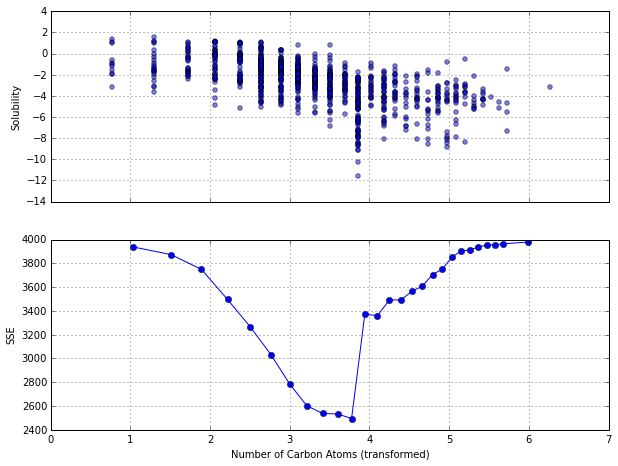Use the regression tree approach, the optimal split point for this variable is 3.78. The reduction in the $SSE$ associated with this split is compared to the optimal valeus for all the other predictors and the split corresponding to the absolute minimum error is used to form subsets $S_1$ and $S_2$. After considering all other variables, this variable was chosen to be the best.

If the process were stopped at this point, all samples with values for this predictor less than 3.78 would be predicted to be 1.84 (the average of the solubility results for these samples) and samples above the splits all have a predicted value for -4.49:

if the number of carbon atoms >= 3.78 then Solubility = -4.49
else Solubility = -1.84
In :
s1 = np.where(trainX['NumCarbon'] <= 3.7768)
s2 = np.where(trainX['NumCarbon'] > 3.7768)
y = [trainY.values[s1, 0], trainY.values[s2, 0]]

plt.boxplot(y)
plt.ylabel('Solubility')
plt.xticks([1, 2], ['<= 3.7768', '> 3.7768'])

Out:
([<matplotlib.axis.XTick at 0x10e289210>,
<matplotlib.axis.XTick at 0x10e292e10>],
<a list of 2 Text xticklabel objects>)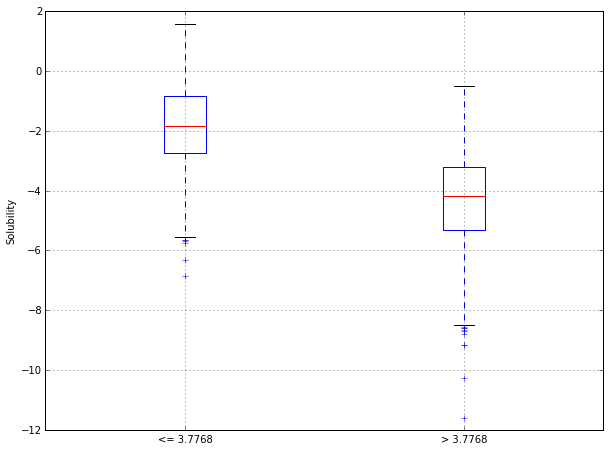In practice, the process then continues within sets $S_1$ and $S_2$ until the number of samples in the splits falls below some threshold. This would conclude the tree growing step.

When the predictor is continuous, the process for finding the optimal split point is straightforward since the data can be ordered in a natural way. Binary predictors are also easy to split, because there is only one possible split point. However, when a predictor has more than two categories, the process for finding the optimal split point can take a couple of justifiable paths.

Once the full tree has been grown, the tree may be very large and is likely to over-fit the training set. The tree is then pruned back to a potentially smaller depth. The goal of this process is to find a "right-sized tree" that has the smallest error rate. To do this, we penalize the error rate using the size of tree: $$\text{SSE}_{C_p} = \text{SSE} + C_p \times (\# \text{Terminal Nodes}),$$ where $C_p$ is called the complexity parameter. For a specific value of the complexity parameter, we find the smallest pruned tree that has the lowest penalized error rate. As with other regularization methods previously discussed, smaller penalties tend to produce more complex models, which, in this ccase, result in larger trees.

To find the best pruned tree, we use cross-validation approaches to evaluate the data across a sequence of $C_p$ values. One can also consider one-standard-error rule to optimize criteria for identifying the simplest tree: find the smallest error tree that is within one standard error of the tree with smallest absolute error.

Note that, unfortunately, sklearn current does not support pruning methods such as the complexity parameter. Instead, a couple of optional parameters, i.e.,

• 'max_depth': the maximum depth of the tree.
• 'min_samples_split': the minimum number of samples required to split an internal node.
• 'min_samples_leaf': the minimum number of samples required to be at a leaf node.

A preferred parameter to specify is the 'min_samples_split' since it directly prevents overfitting.

In :
from sklearn.tree import DecisionTreeRegressor
from sklearn.grid_search import GridSearchCV
from sklearn.cross_validation import ShuffleSplit

cv = ShuffleSplit(trainX.shape, n_iter=10, random_state=3)
treg = DecisionTreeRegressor()
gs_param = {
'min_samples_split': range(2, 100),
}
gs_treg = GridSearchCV(treg, gs_param, cv=cv, scoring="mean_squared_error", n_jobs=-1)
gs_treg.fit(trainX.values, trainY.values[:, 0])

Out:
GridSearchCV(cv=ShuffleSplit(951, n_iter=10, test_size=0.1, random_state=3),
estimator=DecisionTreeRegressor(compute_importances=None, criterion='mse',
max_depth=None, max_features=None, max_leaf_nodes=None,
min_density=None, min_samples_leaf=1, min_samples_split=2,
random_state=None, splitter='best'),
fit_params={}, iid=True, loss_func=None, n_jobs=-1,
param_grid={'min_samples_split': [2, 3, 4, 5, 6, 7, 8, 9, 10, 11, 12, 13, 14, 15, 16, 17, 18, 19, 20, 21, 22, 23, 24, 25, 26, 27, 28, 29, 30, 31, 32, 33, 34, 35, 36, 37, 38, 39, 40, 41, 42, 43, 44, 45, 46, 47, 48, 49, 50, 51, 52, 53, 54, 55, 56, 57, 58, 59, 60, 61, 62, 63, 64, 65, 66, 67, 68, 69, 70, 71, 72, 73, 74, 75, 76, 77, 78, 79, 80, 81, 82, 83, 84, 85, 86, 87, 88, 89, 90, 91, 92, 93, 94, 95, 96, 97, 98, 99]},
pre_dispatch='2*n_jobs', refit=True, score_func=None,
scoring='mean_squared_error', verbose=0)
In :
# calculate RMSE for all candidates
def gs_rmse(scores):
'''Calcuate RMSE for a GridSearchCV grid_scores_ object.'''
rmse = []
for dx, v in enumerate(scores):
rmse.append([v['min_samples_split'], np.sqrt(-v), np.std(np.sqrt(-v))])
return np.array(rmse)

gs_treg_rmse = gs_rmse(gs_treg.grid_scores_)

In :
plt.plot(gs_treg_rmse[:,0], gs_treg_rmse[:,1], c='b')
plt.xlabel('\'min_samples_split\'')
plt.ylabel('RMSE')

Out:
<matplotlib.text.Text at 0x110e61b10>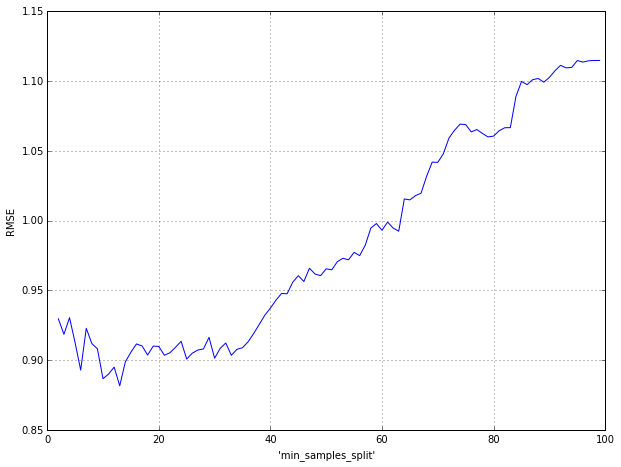In :
# use the one-standard-error rule
thres_rmse = np.min(gs_treg_rmse[:,1]) + gs_treg_rmse[np.argmin(gs_treg_rmse[:,1]), 2]

for idx, i in reversed(list(enumerate(gs_treg_rmse))):
if i < thres_rmse:
best_param = i
break

print "The best parameter: 'min_samples_split' = {0}.".format(best_param)

The best parameter: 'min_samples_split' = 51.0.


This particular tree methodology can also handle missing data. When building the tree, missing data are ignored. For each split, a variety of alternatives (called surrogate splits) are evaluated. A surrogate split is one whose results are similar to the original split actually used in the tree. If a surrogate split approximates the original split well, it can be used when the predictor data associated with the original split are not available.

Once the tree has been finalized, we begin to assess the relative importance of the predictors to the outcome. One way to compute an aggregate measure of importance is to keep track of the overall reduction in the optimization criteria, e.g., SSE. Intuitively, predictors that appear higher in the tree or those that appear multiple times in the tree will be more important than predictors that occur lower in the tree or not at all.

In :
# the important values for the 16 predictors
treg_best = DecisionTreeRegressor(min_samples_split=53)
treg_best.fit(trainX.values, trainY.values[:, 0])

def viz_importance(scores, num_predictors = 16, predictor_names = trainX.columns):
'''Visualize the relative importance of predictors.'''
idx = np.argsort(scores)[-num_predictors:]
names = predictor_names[idx]
importances = scores[idx]

y_pos = np.arange(num_predictors)
plt.barh(y_pos, importances, align='center', alpha=0.4)
plt.yticks(y_pos, names)
plt.xlabel('Importance')

viz_importance(treg_best.feature_importances_)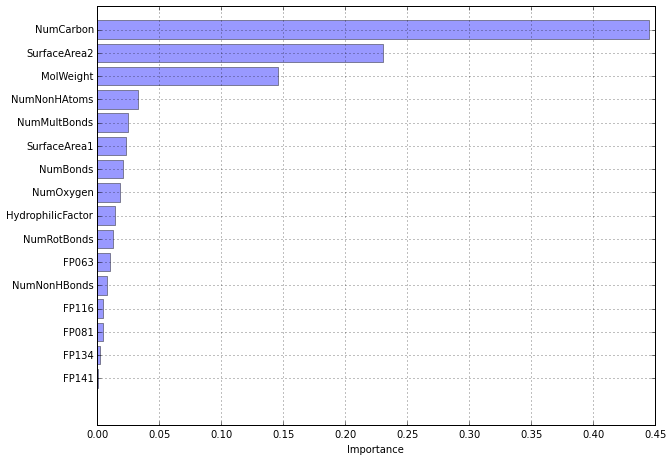An advantage of tree-based models is that, when the tree is not large, the model is simple and interpretable. Tree models intrinsically conduct feature selection; if a predictor is never used in a split, the prediction equation is independent of these data. This advantage is weakened when there are highly correlated predictors. If two predictors are extremely correlated, the choice of which to use in a split is somewhat random. For example, the two surface area predictors have an extremely high correlation (0.96) and each is used in the tree shown in the above figure. It is possible that the difference between these predictors is strongly driving the choice between the two, but it is more likely to be due to small, random differences in the variables. Because of this, more predictors may be selected than actually needed. In addition, the variable importance value for each variable decreases.

While trees are highly interpretable and easy to compute, they do have some noteworthy disadvantages. First, single regression trees are more likely to have sub-optimal predictive performance compared to other modeling approaches. This partly due to the simplicity of the model. By construction, tree models partition the data into rectangular regions of the predictor space. If the relationship between predictors and the outcome is not adequately described by these rectangles, then the predictive performance of a tree will not be optimal. Also, the number of possible predicted outcomes from a tree is finite and is determiend by the number of terminal nodes. This limitation is unlikely to capture all of the nuances of the data.

An additional disadvantage is that an individual tree tends to be unstable. If the data are slightly altered, a completely different set of splits might be found (i.e., the model variance is high). While this is a disadvantage, ensemble methods exploit this characteristic to create models that tend to have extremely good performance.

Finally, these trees suffer from selecion bias: predictors with a higher number of distinct values are favored over more granular predictors. Also, as the number of missing values increases, the selection of predictors becomes more biased.

It is worth noting that the variable importance scores in the above example show that the model tends to rely more on continuous (i.e., less granular) predictors than the binary fingerprints. This could be due to the selection bias or the content of the variables.

## 8.2 Regression Model Trees¶

One limitation of simple regression trees is that each terminal node uses the average of the training set outcomes in that node for prediction. As a consequence, these models may not do a good job predicting samples whose true outcomes are extremely high or low.

One approach to dealing with this issue is to use a different estimator in the terminal nodes. Here we focus on the model tree approach called M5, which is similar to regression trees except:

• The splitting criterion is different.
• The terminal nodes predict the outcome using a linear model (as opposed to the simple average).
• When a sample is predicted, it is often a combination of the predictions from different models along the same path through the tree.

The main implementation of this technique is a "rational reconstruction" of this model called M5, which is included in the Weka software package.

Like simple regression trees, the initial split is found using an exhaustive search over the predictors and training set samples, but, unlike those models, the expected reduction in the node's error rate is used. Let $S$ denote the entire set of data and let $S_1, \cdots, S_p$ represent the $P$ subsets of the data after splitting. The split criterion would be $$\text{reduction} = \text{SD}(S) - \sum_{i=1}^p {n_i \over n} \times \text{SD}(S_i),$$ where $\text{SD}$ is the standard deviation and $n_i$ is the number of samples in partition $i$. This metric determines if the total variation in the splits, weighted by sample size, is lower than in the presplit data. The split that is associated with the largest reduction in error is chosen and a linear model is created within the partitions using the split variable in the model. For subsequent splitting iterations, this process is repeated: an initial split is determined and a linear model is created for the partition using the current split variable and all others that preceded it. The error associated with each linear model is used in place of $\text{SD}(S)$ to determine the expected reduction in the error rate for the next split. The tree growing process continues along the branches of the tree until there are no further improvements in the error rate or there are not enough samples to continue the process. Once the tree is fully grown, there is a linear model for every node in the tree.

Once the complete set of linear models have been created, each undergoes a simplification procedure to potentially drop some of the terms. For a given model, an adjusted error rate is computed. First, the absolute differences between the observed and predicted data are calculated then multiplied by a term that penalizes models with large number of parameters: $$\text{Adjusted Error Rate} = {n^{*}+p \over n^{*}-p} \sum_{i=1}^{n^{*}} | y_i - \hat{y}_i|,$$ where $n^{*}$ is the number of training set data points that were used to build the model and $p$ is the number of parameters. Each model term is dropped and the adjusted error rate is computed. Terms are dropped from the model as long as the adjusted error rate decreases. In some cases, the linear model may be simplified to having only an intercept. This procedure is independently applied to each linear model.

Model trees also incorporate a type of smoothing to decrease the potential for over-fitting. The technique is based on the "recursive shrinking" methodology. When predicting, the new sample goes down the appropriate path of the tree, and moving from the bottom up, the linear models along that path are combined. That is, $$\hat{y}_{(p)} = {n_{(k)} \hat{y}_{(k)} + c\hat{y}_{(p)} \over n_{(k)} + c}$$, where $\hat{y}_{(k)}$ is the prediction from the child note, $n_{(k)}$ is the number of training set data points in the child node, $\hat{y}_{(p)}$ is the prediction from the parent node, and $c$ is a constant with a default value of 15. Once this combined prediction is calculated, it is similarly combined with the next mode along the tree (the next parent node) and so on. Note that the smoothing equation is a relatively simple linear combination of models.

This type of smoothing can have a significant positive effect on the model tree when the linear models across nodes are very different. There are several possible reasons that the linear models may produce very different predictions. First, the number of training set samples that are available in a node will decrease as new splits are added. This can lead to nodes which model very different regions of the training set and, thus, produce very different linear models. This is especially true for small training sets. Secondly, the linear models derived by the splitting process may suffer from significant collinearity. Suppose two predictors in the training set have an extremely high correlation with one another. In this case, the algorithm may choose between the two predictors randomly. If both predictors are eventually used in splits and become candidates for the linear models, there would be two terms in the linear model for effectively one piece of information, which can lead to substantial instability in the model coefficients. Smoothing using several models can help reduce the impact of any unstable linear models.

Once the tree is fully grown, it is pruned back by finding inadequate sub-trees and removing them. Starting at the terminal nodes, the adjusted error rate with and without the sub-tree is computed. If the sub-tree does not decrease the adjusted error-rate, it is pruned from the model. This process is continued until no more sub-trees can be removed.

## 8.3 Rule-Based Models¶

A rule is defined as a distinct path through a tree. For the tree, a new sample can only travel down a single path through the tree defined by these rules. The number of samples affected by a rule is called its coverage.

In addition to the pruning algorithms described before, the complexity of the model tree can be further reduced by either removing entire rules or removing some of the conditions that define the rule.

One approach to creating rules from model trees uses the "separate and conquer" strategy. This procedure derives rules from many different model trees instead of from a single tree. First, an initial model tree is created (it is recommneded to use unsmoothed model trees). However, only the rule with the largest coverage is saved from this model. The samples covered by the rule are removed from the training set and another model tree is created with the remaining data. Again, only the rule with the maximum coverage is retained. This process repeats until all the training set data have been covered by at least one rule. A new sample is preducted by determining which rule(s) it falls under then applies the linear model associated with the largest coverage.

## 8.4 Bagged Trees¶

In the 1990s, ensemble techniques (methods that combine many models' predictions) began to appear. Bagging, short for bootstrap aggregation, was one of the earliest developed ensemble techniques. Bagging is a general approach that uses bootstrapping in conjunction with any regression or classification model to construct a ensemble. The model is fairly simple in structure and consists of the steps outlined below.

for i = 1 to m do
Generate a bootstrap sample of the original data
Train an unprunned tree model on this sample
end

Each model in the ensemble is then used to generate a prediction for a new sample and these $m$ predictions are averaged to give the bagged model's prediction.

Bagging models provide several advantages over models that are not bagged. First, bagging effectively reduces the variance of a prediction through its aggregation process. For models that produce an unstable prediction, like regression tree, aggregating over many versions of the training data actually reduces the variance in the prediction and, hence, makes the prediction more stable. If the goal of the modeling effort is to find the best prediction, then bagging has a distinct advantage.

Bagging stable, lower variance models like linear regression and MARS, on the other hand, offers less imrpovement in the predictive performance.

In :
# use two datasets to illustrate the performance of bagging
solu_trainX = pd.read_csv('../datasets/solubility/solTrainXtrans.csv').values[:, 1:]
solu_trainY = pd.read_csv('../datasets/solubility/solTrainY.csv').values[:, 1]
solu_testX = pd.read_csv('../datasets/solubility/solTestXtrans.csv').values[:, 1:]
solu_testY = pd.read_csv('../datasets/solubility/solTestY.csv').values[:, 1]

concre = pd.read_csv('../datasets/concrete/concrete.csv').drop('Unnamed: 0', 1)
concreX = concre.drop('CompressiveStrength', 1)
concreY = concre['CompressiveStrength']

from sklearn.cross_validation import train_test_split
concre_trainX, concre_testX, concre_trainY, concre_testY = train_test_split(concreX, concreY, test_size = 0.2)

In :
# some basic setups
def boostrap_sample(X, Y):
'''Generate a boostrap sample of the original data.'''
bsample = np.random.choice(range(len(Y)), len(Y), replace=True)
bs_X = X[bsample]
bs_Y = Y[bsample]
return bs_X, bs_Y

def test_pred(trainX, trainY, testX, model):
'''Generate a test set prediction based on the given model.'''
reg = model
reg.fit(trainX, trainY)
return reg.predict(testX)

def test_rmse(Y, pred_Y):
'''Calculate RMSE based on the test set prediction.'''
return np.sqrt(np.mean((Y - pred_Y)**2))

In :
# bagging: tree vs linear regression vs MARS
from sklearn.tree import DecisionTreeRegressor
from sklearn.linear_model import LinearRegression
from pyearth import Earth

np.random.seed(3)

tree_solu_rmse = []
tree_concre_rmse = []
lm_solu_rmse = []
lm_concre_rmse = []
mars_solu_rmse = []
mars_concre_rmse = []

for i in range(0, 50, 2):
# generate a boostrap sample
bs_solu_trainX, bs_solu_trainY = boostrap_sample(solu_trainX, solu_trainY)
bs_concre_trainX, bs_concre_trainY = boostrap_sample(concre_trainX, concre_trainY)

# train a model
tree_solu = test_pred(bs_solu_trainX, bs_solu_trainY, solu_testX, DecisionTreeRegressor())
lm_solu = test_pred(bs_solu_trainX, bs_solu_trainY, solu_testX, LinearRegression())
mars_solu = test_pred(bs_solu_trainX, bs_solu_trainY, solu_testX, Earth())
tree_concre = test_pred(bs_concre_trainX, bs_concre_trainY, concre_testX, DecisionTreeRegressor())
lm_concre = test_pred(bs_concre_trainX, bs_concre_trainY, concre_testX, LinearRegression())
mars_concre = test_pred(bs_concre_trainX, bs_concre_trainY, concre_testX, Earth())

# aggregate prediction
if i != 0:
tree_solu_agg = np.vstack([tree_solu_agg, tree_solu])
lm_solu_agg = np.vstack([lm_solu_agg, lm_solu])
mars_solu_agg = np.vstack([mars_solu_agg, mars_solu])
tree_concre_agg = np.vstack([tree_concre_agg, tree_concre])
lm_concre_agg = np.vstack([lm_concre_agg, lm_concre])
mars_concre_agg = np.vstack([mars_concre_agg, mars_concre])
else: # if agg not defined
tree_solu_agg = tree_solu
lm_solu_agg = lm_solu
mars_solu_agg = mars_solu
tree_concre_agg = tree_concre
lm_concre_agg = lm_concre
mars_concre_agg = mars_concre

# calculate rmse
tree_solu_rmse.append(test_rmse(solu_testY, np.mean(tree_solu_agg, 0)))
lm_solu_rmse.append(test_rmse(solu_testY, np.mean(lm_solu_agg, 0)))
mars_solu_rmse.append(test_rmse(solu_testY, np.mean(mars_solu_agg, 0)))
tree_concre_rmse.append(test_rmse(concre_testY, np.mean(tree_concre_agg, 0)))
lm_concre_rmse.append(test_rmse(concre_testY, np.mean(lm_concre_agg, 0)))
mars_concre_rmse.append(test_rmse(concre_testY, np.mean(mars_concre_agg, 0)))


The test set performance based on RMSE is plooted by number of bagging iterations.

In :
fig, (ax1, ax2) = plt.subplots(nrows = 2, sharex = True)

# solubility data
l1, l2, l3 = ax1.plot(range(0, 50, 2)[1:], tree_solu_rmse[1:], '-o',
range(0, 50, 2)[1:], lm_solu_rmse[1:], '-x',
range(0, 50, 2)[1:], mars_solu_rmse[1:], '-^')
ax1.set_title('Solubility')

# concrete date
ax2.plot(range(0, 50, 2)[1:], tree_concre_rmse[1:], '-o',
range(0, 50, 2)[1:], lm_concre_rmse[1:], '-x',
range(0, 50, 2)[1:], mars_concre_rmse[1:], '-^')
ax2.set_title('Concrete')

fig.legend((l1, l2, l3), ('Tree', 'Linear Regression', 'MARS'), loc='upper center', ncol=3, frameon=False)
fig.text(0.5, 0.06, 'Number of Bagging Iterations', ha='center', va='center')
fig.text(0.06, 0.5, 'RMSE', va='center', rotation='vertical')

Out:
<matplotlib.text.Text at 0x103778c50>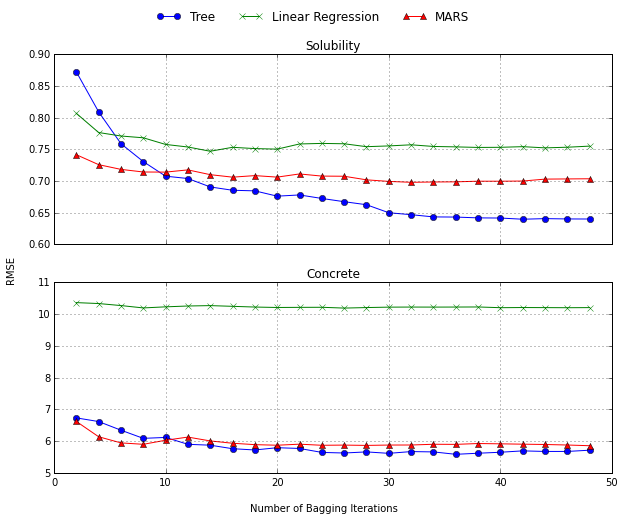Linear regression and MARS are least improved throughout the ensemble, while the predictions for regression trees are dramatically improved.

As a further demonstration of bagging's ability to reduce the variance of a model's prediction, consider a set of simulated sin waves. For this illustration, 20 sin waves were simulated, and, for each data set, regression trees and MARS model, as well as bagged regressions and MARS models (each with 50 model iterations), were computed.

In :
# simulated 20 sin waves
np.random.seed(3)

X = np.zeros([20, 100])
Y = np.zeros([20, 100])
for i in range(20):
X[i,:] = np.sort(np.random.uniform(2, 10, 100))
Y[i,:] = np.sin(X[i,:]) + np.random.normal(0, 0.2, 100)

In :
def model_pred(x, y, model):
'''Fit model and generate predictions.'''
reg = model
reg.fit(x, y)
return reg.predict(x)

def bagg_model_pred(x, y, model, niter=50):
'''Fit bagging model with 50 iterations and generate predictions.'''
for i in range(niter):
bs_x, bs_y = boostrap_sample(x, y)
bs_order = np.argsort(bs_x, axis=0)[:,0]
pred = model_pred(bs_x[bs_order], bs_y[bs_order], model)
if i != 0:
pred_agg = np.vstack([pred_agg, pred])
else:
pred_agg = pred
return np.mean(pred_agg, 0)

In :
# build models for each sin wave and make predictions
tree_pred = []
mars_pred = []
bagg_tree_pred = []
bagg_mars_pred = []

for i in range(20):
x = X[i,:][:,np.newaxis]
y = Y[i,:]

tree_pred.append(model_pred(x, y, DecisionTreeRegressor(max_depth=3)))
mars_pred.append(model_pred(x, y, Earth()))
bagg_tree_pred.append(bagg_model_pred(x, y, DecisionTreeRegressor(max_depth=3), 50))
bagg_mars_pred.append(bagg_model_pred(x, y, Earth(), 50))

In :
fig, axarr = plt.subplots(2, 2)

x_test = np.arange(2.0, 10.0, 0.01)

# tree
axarr[0, 0].plot(x_test, np.sin(x_test), 'r')
for i in range(20):
axarr[0, 0].plot(X[i,:], tree_pred[i], 'black', alpha=0.3)
axarr[0, 0].set_title('Tree')
axarr[0, 0].set_ylim([-1.5, 1.5])

# bagged tree
axarr[1, 0].plot(x_test, np.sin(x_test), 'r')
for i in range(20):
axarr[1, 0].plot(X[i,:], bagg_tree_pred[i], 'black', alpha=0.3)
axarr[1, 0].set_title('Bagged Tree')
axarr[1, 0].set_ylim([-1.5, 1.5])

# MARS
axarr[0, 1].plot(x_test, np.sin(x_test), 'r')
for i in range(20):
axarr[0, 1].plot(X[i,:], mars_pred[i], 'black', alpha=0.25)
axarr[0, 1].set_title('MARS')
axarr[0, 1].set_ylim([-1.5, 1.5])

# bagged MARS
axarr[1, 1].plot(x_test, np.sin(x_test), 'r')
for i in range(20):
axarr[1, 1].plot(X[i,:], bagg_mars_pred[i], 'black', alpha=0.25)
axarr[1, 1].set_title('Bagged MARS')
axarr[1, 1].set_ylim([-1.5, 1.5])

Out:
(-1.5, 1.5)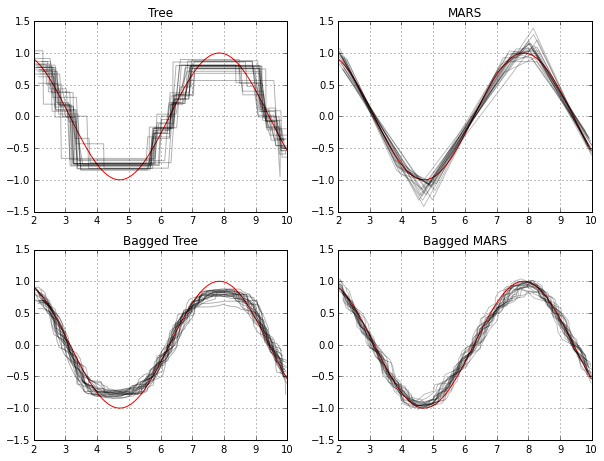The red lines in the panels show the true trend while the multiple black lines show the predictions for each model. Note that the Tree panel has more noise around the true sin curve than the MARS model, which only shows variation at the change of points of the pattern. This illustrates the high variance in the regression tree due to model instability. The bottom panels of the figure show the results for bagged models. The variation around the true curve is greatly reduced for regression trees, and, for MARS, the variation is only reduced around the curvilinear portions on the pattern.

Variance/bias tradeoff: Bagging improves the RMSE of the fitted models by reducing the variance, but not the biases.

Another advantage of bagging models is that they can provide their own internal estimate of predictive performance that correlates well with either cross-validation estimates or test set estimates. Here's why: when constructing a bootstrap sample for each model in the ensemble, certain samples are left out. These samples are called out-of-bag, and they can be used to assess the predictive performance courtesy of that specific model since they were not used to build the model. Hence, every model in the ensemble generates a measure of predictive performance courtesy of the out-of-bag samples. The average of the out-of-bag performance metrics can then be used to gauge the predictive performance of the entire ensemble, and this value usually correlates well with the assessment of predictive performance we can get with either cross-validation or from a test set. This error estimate is usually referred to as the out-of-bag estimate.

In its basic form, the user has one choice to make for bagging: the number of bootstrap samples to aggregate, m. Often we see an exponential decrease in predictive improvement as the number of iterations increase; the most improvement in prediction performance is obtained with a small number of trees (m < 10).

In :
# display cross-validated predictive performance (RMSE) for varing number of bootstraped samples
def bagg_rmse(trainX, trainY, testX, testY, num_bagged, model):
'''Calculate RMSE on test set for bagged models.'''
for i in range(num_bagged):
bs_x, bs_y = boostrap_sample(trainX, trainY)
reg = model
reg.fit(bs_x, bs_y)
pred = reg.predict(testX)
if i != 0:
pred_agg = np.vstack([pred_agg, pred])
else:
pred_agg = pred
pred_avg = np.mean(pred_agg, 0)
return np.sqrt(np.mean((pred_avg - testY)**2))

In :
from sklearn.cross_validation import ShuffleSplit

cv = ShuffleSplit(solu_trainX.shape, n_iter=10, random_state=3)

cv_rmse = []

for num_bagged in np.logspace(0, 2, num=7).astype(int):

temp_rmse = []

for train_indices, test_indices in cv:
trainX = solu_trainX[train_indices]
trainY = solu_trainY[train_indices]
testX = solu_trainX[test_indices]
testY = solu_trainY[test_indices]

temp_rmse.append(bagg_rmse(trainX, trainY, testX, testY, num_bagged,
DecisionTreeRegressor(max_depth=5)))
cv_rmse.append(temp_rmse)

In :
plt.errorbar(np.logspace(0, 2, num=7).astype(int),
np.mean(cv_rmse, 1), yerr = np.std(cv_rmse, 1))
plt.xlim(-2, 102)
plt.show()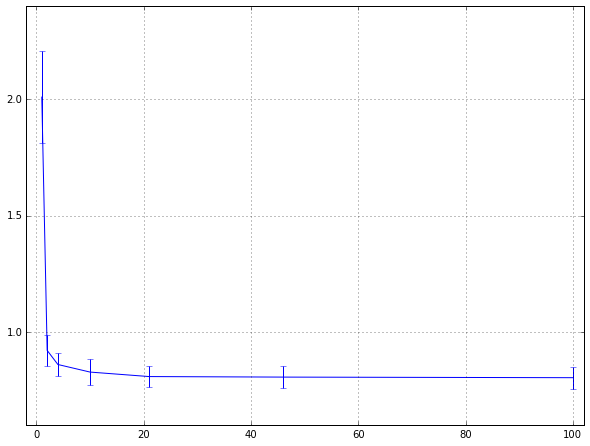Notice predictive performance improves through ten trees and then tails off with very limited improvment beyond that point. In our experience, if performance is not at an acceptable level after 50 bagging iterations, then we suggest trying other more powerfully predictive ensemble methods such as random forests and boosting.

Although bagging usually improves predictive performance for unstable models, there are a few caveats. First, computational costs and memory requirements increase as the number of bootstrap samples increases. This disadvantage can be mostly mitigated if the modeler has access to parallel computing because the bagging process can be easily parallelized. Another disadvantage to this approach is that a bagged model is much less interpretable than a model that is not bagged. However, measures of variable importance can be constructed by combining measures of importrance from the individual models across the ensemble.

## 8.5 Random Forests¶

Bagging trees (or any high variance, low bias technique) improves predictive performance over a single tree by reducing variance of the prediction. Generating bootstrap samples introduces a random component into the tree building process, which induces a distribution of trees, and therefore also a distribution of predicted values for each sample. The trees in bagging, however, are not completely independent of each other since all of the original predictors are considered at every split of every tree. One can imagine that if we start with a sufficiently large number of original samples and a relationship between predictors and response that can be adequately modeled by a tree, then trees from different bootstrap samples may have similar structures to each other due to the underlying relationship. This characteristic is known as tree correlation and prevents bagging from optimally reducing variance of the predicted values. Reducing correlation among trees, known as de-correlating trees, is then the next logical step to improving the performance of bagging.

From a statistical perspective, reducing correlation among predictors can be done by adding randomness to the tree construction process. A general random forests algorithm for a tree-based model can be implemented as below.

Select the number of models to build, m
for i = 1 to m do
Generate a bootstrap sample of the original data
Train a tree model on this sample
for each split do
Randomly select k (<p) of the original predictors
Select the best predictor among the k predictors and partition the data
end
Use typical tree model stopping criteria to determine when a tree is complete (but do not prune)
end

Random forests' tuning parameter is the number of randomly selected predictors, k, to choose from at each split, and is commonly referred to as $m_{try}$. In the regression context, it is suggested to set $m_{try}$ to be one-third of the number of predictors. For the purpose of tuning the $m_{try}$ parameter, since random forests is computationally intensive, we suggest starting with five values of k that are somewhat evenly spaced across the range from 2 to p. The practioner must also specify the number of trees for the forest. It is proved that random forests is protected from over-fitting. Practically speaking, the larger the forest, the more computational burden we will incur to train and build the model. As a starting point, we suggest using at least 1000 trees.

It is shown that the linear combination of many independent learners reduces the variance of the overall ensemble relative to any individual learner in the ensemble. A random forest model achieves this variance reduction by selecting strong, complex learners that exhibit low bias. This ensemble of many independent, strong learners yields an improvement in error rates. Because each learner is selected independently of all previous learners, random forests is robust to a noisy response. At the same time, the independence of learners can underfit data when the response is not noisy.

Compared to bagging, random forests is more computationally efficient on a tree-by-tree basis since the tree building process only needs to evaluate a fraction of the original predictors at each split, although more trees are usually required by random forests. Combining this attribute with the ability to parallel process tree building makes random forests more computationally efficient than boosting.

Tree was used as the base learner in random forests, as well as 10-fold cross-validation and out-of-bag validation, to train models on the solubility data. The $m_{try}$ parameter was evaluated at ten values from 10 to 228.

In :
from sklearn.ensemble import RandomForestRegressor
from sklearn.metrics import mean_squared_error

cv = ShuffleSplit(solu_trainX.shape, n_iter=10, random_state=3)

cv_rmse = []
oob_rmse = []
for m_try in range(10, 228, 22):
# out-of-bag score
rf = RandomForestRegressor(n_estimators=1000, max_features=m_try, oob_score=True)
rf.fit(solu_trainX, solu_trainY)
oob_rmse.append(np.sqrt(mean_squared_error(solu_trainY, rf.oob_prediction_)))

# cross-validation score
cv_temp_rmse = []
for train_indices, test_indices in cv:
trainX = solu_trainX[train_indices]
trainY = solu_trainY[train_indices]
testX = solu_trainX[test_indices]
testY = solu_trainY[test_indices]

rf = RandomForestRegressor(n_estimators=1000, max_features=m_try)
rf.fit(trainX, trainY)
cv_temp_rmse.append(np.sqrt(mean_squared_error(testY, rf.predict(testX))))
cv_rmse.append(cv_temp_rmse)

In :
plt.plot(range(10, 228, 22), np.mean(cv_rmse, 1), 'o-', label = 'CV score')
plt.plot(range(10, 228, 22), oob_rmse, 'x-', label = 'OOB score')
plt.legend()
plt.xlim(0, 228)
plt.show()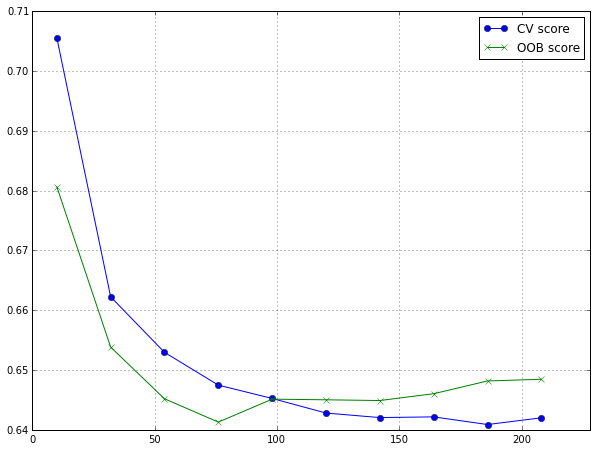Our experience is that the random forest tuning parameter does not have a drastic effect on performance. In these data, the only real difference in the RMSE comes when the smallest value is used (10 in this case). It is often the case that such a small value is not associated with optimal performance. However, we have seen rare examples where small tuning parameter values generate the best results. To get a quick assessment of how well the random forest model performs, the default tuning parameter value for regression ($m_{try} = p/3$) tends to work well. If there is a desire to maximize performance, tuning this value may result in a slight improvment. Also, note that, use the out-of-bag error rate would drastically decrease the computational time to tune random forest models.

The ensemble nature of random forests makes it impossible to gain an understanding of the relationship between the predictors and the response. However, because trees are the typical base learner for this method, it is possible to quantify the impact of predictors in the ensemble. One approach is to randomly permuting the values of each predictor for the out-of-bag sample of one predictor at a tiem for each tree. The difference in predictive performance between the non-permuted sample and the permuted sample for each predictor is recorded and aggregated across the entire forest. Another approach is to measure the improvement in the node purity based on the performance metric for each predictor at each occurence of that predictor across the forest. These individual improvement values for each predictor are then aggregated across the forest to determine the overall importance for the predictor.

Although this strategy to determine the relative influence of a predictor is very different from the approach for single regression tree, it suffers from the same limitations related to bias. Also, the correlations between predictors can have a significant impact on the importance values. Also, the $m_{try}$ tuning parameter has a serious effect on the importance values.

Another impact of between-predictor correlations is to dilute the importances of key predictors. For example, suppose a critical predictor had an importance of X. If another predictor is just as critical but is almost perfectly correlated as the first, the importance of these two predictors will be roughly X/2. If three such predictors were in the model, the values would further decrease to X/3 and so on.

In :
rf = RandomForestRegressor(n_estimators=1000, max_features=120)
rf.fit(solu_trainX, solu_trainY)
viz_importance(rf.feature_importances_)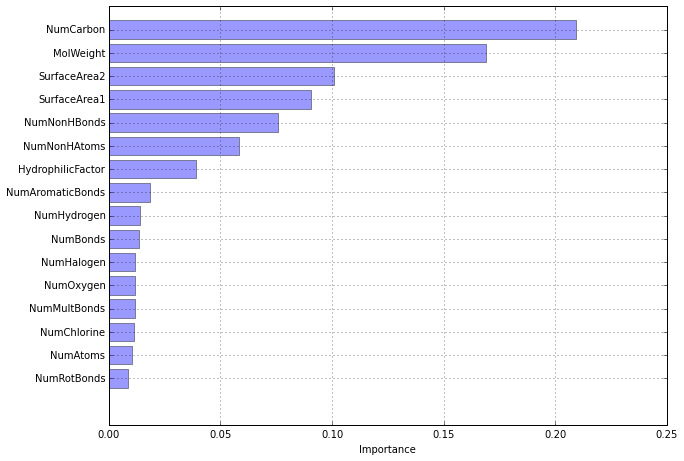Contrasting random forest importance results to a singe tree, we see many important predictors are in common. However, the importance ordering are much different. These differences should not be disconerning; rather they emphasize that a single tree's greediness prioritizes predictors differently than a random forest.

## 8.6 Boosting¶

Boosting models were originally developed for classification problems and were later extended to the regression setting. Boosting, especially in the form of AdaBoost algorithm, was shown to be a powerful predictive tool, usually outperforming any individual model. The AdaBoost algorithm clearly worked, and after its successful arrival, several reseachers connected the AdaBoost algorithm to statistical concepts of loss functions, additive modeling, and logistic regression and showed that boosting can be interpreted as a forward stagewise additive model that minimize exponential loss. This fundamental understanding of boosting led to a new view of boosting that facilitated several algorithmic generalizations to classification problems. Moreover, this new perspective also enabled the method to be extended to regression problem.

The "gradient boosting machines" is a method stemmed from boosting's statistical framework, which emcompassed both classification and regression. The basic principles of gradient boosting are as follows: given a loss function (e.g., squared error for regression) and a weak learner (e.g., regression trees), the algorithm seeks to find an additive model that minimizes the loss function. The algorithm is typically initialized with the best guess of the response (e.g., the mean of the response in regression). The gradient (e.g., residual) is calculated, and a model is then fit to the residuals to minimize the loss function. The current model is added to the previous model, and the procedure continues for a use-specified number of iterations.

As described throughout this text, any modeling technique with tuning parameters can produce a range of predictive ability -- from weak to strong. Because boosting requires a weak learner, almost any technique with tuning parameters can be made into a weak learner. Tree, as it turns out, make an excellent base learner for boosting for several reasons. First, they have the flexibility to be weak learners by simply restricting their depth. Second, separate trees can be easily added together, much like individual predictors can be added together in a regression model, to generate a prediction. And third, trees can be generated very quickly. Hence, results from individual trees can be directly aggregated, thus making them inherently suitable for an additive modeling process.

When regression trees are used as the base learner, simple gradient boosting for regression has two tuning parameters: tree depth and number of iterations. Tree depth in this context is also known as interaction depth, since each subsequential split can be thought of as a higher-level interaction term with all of the other previous split predictors. If squared error is used as the loss function, then a simple boosting algorithm using these tuning parameters is listed below.

Select tree depth, D, and number of iterations, K
Compute the average response, $\bar{y}$, and use this as the initial predicted value for each sample
for k = 1 to K do
Compute the residual, the difference between the observed value and the current predicted value, for each sample
Fit a regression tree of depth, D, using the residuals as the response
Predict each sample using the regression tree fit in the previous step
Update the predicted value of each sample by adding the previous iteration's predicted value to the predicted value
generated in the previous step
end

Clearly, this version of boosting has similarities to random forests: the final prediction is based on an ensemble of models, and trees are used as the base learner. However, the way the ensembles are constructed differs substantially between each model. In random forests, all trees are created independently, each tree is created to have maximum depth, and each tree contributes equally to the final model. The trees in boosting, however, are dependent on past trees, have minimum depth, and contribute unequally to the final model. Despite these differences, both random forests and boosting offer competitive predictive performance. Computation time for boosting is often greater than for random forests, since random forests can be easily parallel processed given that the trees are created independently.

The gradient boosting machine could be susceptible to over-fitting, since the learner employed -- even in its weakly defined learning capacity -- is tasked with optimally fitting the gradient. This means that boosting will select the optimal learner at each stage of the algorithm. Despite using weak learners, boosting still employs the greedy strategy of choosing the optimal weak learner at each stage. Although this strategy generates an optimal solution at the current stage, it has the drawbacks of not finding the optimal global model as well as over-fitting the training data. A remedy for greediness is to constrain the learning process by employing regularization, or shrinkage. In the above algorithm, instead of adding the predicted value for a sample to previous iteration's predicted value, only a fraction of the current predicted value is added to the previous iteration's predicted value. This fraction is commonly referred to as the learning rate and is parameterized by the symbol, $\lambda$. THis parameter can take values between 0 and 1 and becomes another tuning parameter for the model. Small values of the learning parameter ($<$0.01) works best, but the value of the parameter is inversely proportional to the computation time required to find an optimal model, because more iterations are necessary. Having more iterations also implies that more memory is required for storing the model.

A variation, stochastic gradient boosting, borrows some idea from bagging techniques. It inserts the following step before line within the loop: randomly select a fraction of the training data. The residuals and models in the remaining steps of the current iteration are based only on the sample of data. The fraction of training data used, knownas the bagging fraction, then becomes another tuning parameter for the model. It turns out that this simple modification improved the predictive accuracy of boosting while also reducing the required computational resources.

In :
from sklearn.ensemble import GradientBoostingRegressor
from sklearn.grid_search import GridSearchCV
from sklearn.cross_validation import ShuffleSplit

cv = ShuffleSplit(solu_trainX.shape, n_iter=10, random_state=3)

gs_param = {
'learning_rate': [0.01, 0.1],
'max_depth': range(1, 8, 2),
'n_estimators': range(100, 1001, 50),
'subsample': [0.5],
}
gs_gbr = GridSearchCV(gbr, gs_param, cv=cv, scoring='mean_squared_error', n_jobs=-1)
gs_gbr.fit(solu_trainX, solu_trainY)

Out:
GridSearchCV(cv=ShuffleSplit(951, n_iter=10, test_size=0.1, random_state=3),
estimator=GradientBoostingRegressor(alpha=0.9, init=None, learning_rate=0.1, loss='ls',
max_depth=3, max_features=None, max_leaf_nodes=None,
min_samples_leaf=1, min_samples_split=2, n_estimators=100,
random_state=None, subsample=1.0, verbose=0, warm_start=False),
fit_params={}, iid=True, loss_func=None, n_jobs=-1,
param_grid={'n_estimators': [100, 150, 200, 250, 300, 350, 400, 450, 500, 550, 600, 650, 700, 750, 800, 850, 900, 950, 1000], 'subsample': [0.5], 'learning_rate': [0.01, 0.1], 'max_depth': [1, 3, 5, 7]},
pre_dispatch='2*n_jobs', refit=True, score_func=None,
scoring='mean_squared_error', verbose=0)
In :
scores1 = np.zeros([len(range(100, 1001, 50)), len(range(1, 8, 2))])
scores2 = np.zeros([len(range(100, 1001, 50)), len(range(1, 8, 2))])

for score in gs_gbr.grid_scores_:
if score['learning_rate'] == 0.01:
idx = (score['n_estimators'] - 100)/50
jdx = score['max_depth']/2
scores1[idx, jdx] = np.sqrt(-score)
else:
idx = (score['n_estimators'] - 100)/50
jdx = score['max_depth']/2
scores2[idx, jdx] = np.sqrt(-score)

In :
fig, (ax1, ax2) = plt.subplots(ncols=2, sharey=True)

for i in range(4):
ax1.plot(range(100, 1001, 50), scores1[:, i], 'x-')
ax1.set_title('shrinkage: 0.01')

for i in range(4):
ax2.plot(range(100, 1001, 50), scores2[:, i], 'x-', label = 'tree depth:' + str(2*i+1))
ax2.set_title('shrinkage: 0.10')
ax2.legend(loc='upper right')

fig.text(0.5, 0.06, '# Trees', ha='center', va='center')
fig.text(0.08, 0.5, 'RMESE (Cross-Validation)', ha='center', va='center', rotation=90)

Out:
<matplotlib.text.Text at 0x114438490>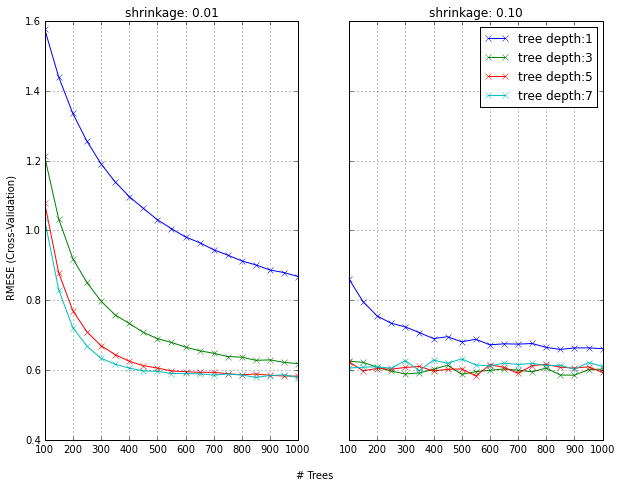The cross-validated RMSE results is presented for boosted trees across tuning parameters of tree depth (1-7), number of trees (100-1000), and shrinkage (0.01 or 0.10); the bagging fraction was fixed at 0.5. The larger value of shrinkage has an impact on reducing RMSE for all choices of tree depth and number of trees. The same pattern holds true for RMSE when shrinkage is 0.1 and the number of trees is less than 300.

Variable importance for boosting is a function of the reduction in squared error. Specifically, the improvement in squared error due to each predictor is summed within each tree in the ensemble (i.e., each predictor gets an improvement value for each tree). The improvement values for each predictor are then averaged across the entire ensemble to yield an overall importance value.

In :
gbr = GradientBoostingRegressor(learning_rate=0.01, max_depth=5, n_estimators=500, subsample=0.5)
gbr.fit(solu_trainX, solu_trainY)
viz_importance(gbr.feature_importances_)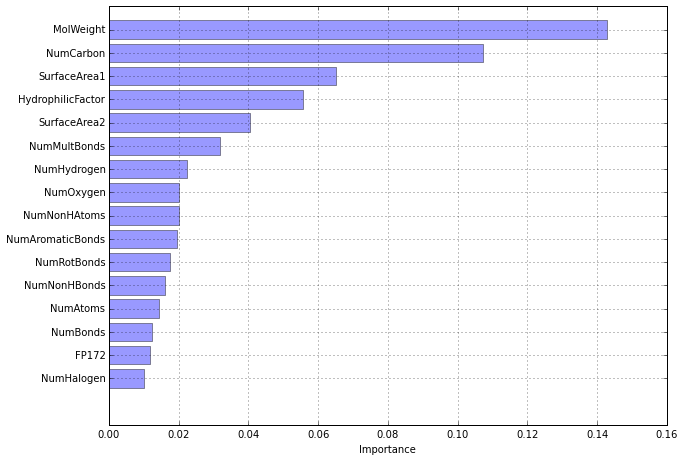The importance profile for boosting has a much steeper importance slope than the one for random forests. This is due to the fact that the trees from boosting are dependent on each other and hence will have correlated structures as the method follows by the gradient. Therefore, many of the same predictors will be selected across the trees, increasing their contribution to the importance metric. Differences between variable importance ordering and magnitude between random forests and boosting should not be disconcerning. Instead, one should consider these as two different perspectives of the data and use each view to provide some understanding of the gross relationships between predictors and the response.